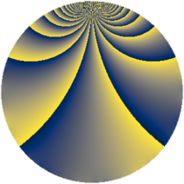# Properties

 Label 3549.2.baLevel $3549$ Weight $2$ Character orbit 3549.ba Rep. character $\chi_{3549}(1013,\cdot)$ Character field $\Q(\zeta_{6})$ Dimension $780$ Sturm bound $970$

# Related objects

## Defining parameters

 Level: $$N$$ $$=$$ $$3549 = 3 \cdot 7 \cdot 13^{2}$$ Weight: $$k$$ $$=$$ $$2$$ Character orbit: $$[\chi]$$ $$=$$ 3549.ba (of order $$6$$ and degree $$2$$) Character conductor: $$\operatorname{cond}(\chi)$$ $$=$$ $$273$$ Character field: $$\Q(\zeta_{6})$$ Sturm bound: $$970$$

## Dimensions

The following table gives the dimensions of various subspaces of $$M_{2}(3549, [\chi])$$.

Total New Old
Modular forms 1028 860 168
Cusp forms 916 780 136
Eisenstein series 112 80 32

## Trace form

 $$780q + 6q^{3} - 364q^{4} + 6q^{9} + O(q^{10})$$ $$780q + 6q^{3} - 364q^{4} + 6q^{9} + 12q^{10} - 6q^{12} - 312q^{16} + 24q^{22} + 310q^{25} + 4q^{30} - 88q^{36} + 36q^{40} - 46q^{42} + 20q^{43} + 14q^{49} - 34q^{51} - 24q^{61} + 512q^{64} - 156q^{66} + 114q^{75} - 18q^{79} - 50q^{81} + 24q^{82} + 48q^{87} - 12q^{88} - 168q^{94} + O(q^{100})$$

## Decomposition of $$S_{2}^{\mathrm{new}}(3549, [\chi])$$ into newform subspaces

The newforms in this space have not yet been added to the LMFDB.

## Decomposition of $$S_{2}^{\mathrm{old}}(3549, [\chi])$$ into lower level spaces

$$S_{2}^{\mathrm{old}}(3549, [\chi]) \cong$$ $$S_{2}^{\mathrm{new}}(273, [\chi])$$$$^{\oplus 2}$$